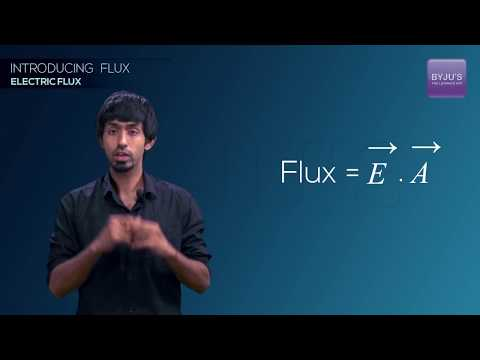Checkout JEE MAINS 2022 Question Paper Analysis : Checkout JEE MAINS 2022 Question Paper Analysis :

# Dimensions of Charge

## Dimensional Formula of Charge

The dimensional formula of charge is given by,

MLTI1

Where,

• M = Mass
• I = Current
• L = Length
• T = Time

### Derivation

Electric Charge (Q) = Electric Current × Time . . . . (1)

The dimensional formula of Current and Time is [M0 L0 TI1] and [M0 L0 T1] respectively . . . . (2)

On substituting equation (2) in equation (1) we get,

Electric Charge = Electric Current × Time

Or, Q = [M0 L0 TI1]

Therefore, the charge is dimensionally represented as [M0 L0 TI1].

## Electric Potential Energy## Electric Flux